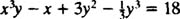## Exact Equations

A first‐order differential equation is one containing a first—but no higher—derivative of the unknown function. For virtually every such equation encountered in practice, the general solution will contain one arbitrary constant, that is, one parameter, so a first‐order IVP will contain one initial condition. There is no general method that solves every first‐order equation, but there are methods to solve particular types.

Given a function f( x, y) of two variables, its total differential df is defined by the equation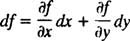Example 1: If f( x, y) = x 2 y + 6 xy 3, thenThe equation f( x, y) = c gives the family of integral curves (that is, the solutions) of the differential equationTherefore, if a differential equation has the form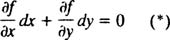for some function f( x, y), then it is automatically of the form df = 0, so the general solution is immediately given by f( x, y) = c. In this case,is called an exact differential, and the differential equation (*) is called an exact equation. To determine whether a given differential equationis exact, use the Test for Exactness: A differential equation M dx + N dy = 0 is exact if and only ifExample 2: Is the following differential equation exact?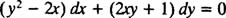The function that multiplies the differential dx is denoted M( x, y), so M( x, y) = y 2 – 2 x; the function that multiplies the differential dy is denoted N( x, y), so N( x, y) = 2 xy + 1. Sincethe Test for Exactness says that the given differential equation is indeed exact (since M y = N x ). This means that there exists a function f( x, y) such thatand once this function f is found, the general solution of the differential equation is simply(where c is an arbitrary constant).

Once a differential equation M dx + N dy = 0 is determined to be exact, the only task remaining is to find the function f ( x, y) such that f x = M and f y = N. The method is simple: Integrate M with respect to x, integrate N with respect to y, and then “merge” the two resulting expressions to construct the desired function f.

Example 3: Solve the exact differential equation of Example 2:First, integrate M( x,y) = y 2 – 2 x with respect to x (and ignore the arbitrary “constant” of integration):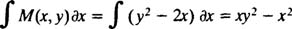Next, integrate N( x,y) = 2 xy + 1 with respect to y (and again ignore the arbitrary “constant” of integration):Now, to “merge” these two expressions, write down each term exactly once, even if a particular term appears in both results. Here the two expressions contain the terms xy 2, – x 2, and y, so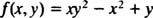(Note that the common term xy 2 is not written twice.) The general solution of the differential equation is f( x,y) = c, which in this case becomes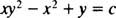Example 4: Test the following equation for exactness and solve it if it is exact: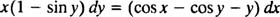First, bring the dx term over to the left‐hand side to write the equation in standard form:Therefore, M( x,y) = y + cos y – cos x, and N ( x, y) = xx sin y.

Now, since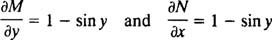the Test for Exactness says that the differential equation is indeed exact (since M y = N x ). To construct the function f ( x,y) such that f x = M and f y N, first integrate M with respect to x:Then integrate N with respect to y:Writing all terms that appear in both these resulting expressions‐ without repeating any common terms–gives the desired function: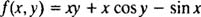The general solution of the given differential equation is thereforeExample 5: Is the following equation exact?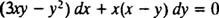Sincebutit is clear that M y N x , so the Test for Exactness says that this equation is not exact. That is, there is no function f ( x,y) whose derivative with respect to x is M ( x,y) = 3 xyf 2 and which at the same time has N ( x,y) = x ( x – y) as its derivative with respect to y.

Example 6: Solve the IVPThe differential equation is exact becauseIntegrating M with respect to x givesand integrating N with respect to y yields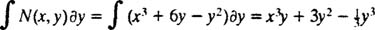Therefore, the function f( x,y) whose total differential is the left‐hand side of the given differential equation is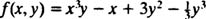and the general solution is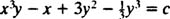The particular solution specified by the IVP must have y = 3 when x = 0; this condition determines the value of the constant c: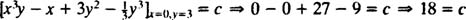Thus, the solution of the IVP is